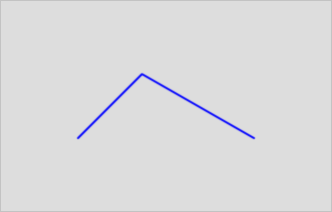This topic describes the ST_SimplifyVW function. This function returns a geometry object representing a simplified version of the input geometry object. This function uses the Visvalingam-Whyatt algorithm.

## Syntax

``geometry  ST_SimplifyVW(geometry  geomA , float  tolerance);``

## Parameters

Parameter Description
geomA The geometry object that you want to specify.
tolerance The tolerance that you want to specify.

## Description

• This function supports simplification operations only on MultiLine objects, MultiPolygon objects, and MultiPoint objects. However, you can use this function to process any geometry objects.
• The simplification operation is performed on the input geometry objects one by one. Therefore, you can use this function to process GeometryCollection objects.
• The returned geometry object may lose its simplicity.
• This function may change the topology of the input geometry object and return an invalid geometry object.

You can use the ST_SimplifyPreserveTopology function to preserve the topology of the input geometry object.

• This function supports 3D objects, and the 3D dimension of the input geometry object affects the returned geometry object.

## Examples

Comparison between the results of the ST_Simplify function and the ST_SimplifyVW function:
``````select g,ST_Simplify(g,0.5),
ST_SimplifyVW(g,0.5)
from (select 'LINESTRING(0 0,2 2,3 1,3.5 1.5,5 0,5.25 0.25,5.5 0)'::geometry as g) as t;``````